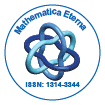# Mathematica EternaOpen Access

ISSN: 1314-3344

## Abstract

### Existence and uniqueness theorems for a class of equations of interaction type between a vibrating structure and a fluid

Marie-ThÃÂ´er`ese Aimar and Abdelkader Intissar

The problems of the interaction between a vibrating structure and a fluid have been studied by many authors, see for example the interesting article  and their references. The principal objective of this work is to investigate the solvability of some problems of interaction between structure and fluid by a mathematical method based upon the analytic semigroups and fractional powers of operators and which can be applied to wider range of physical situations. In this paper, we develop this method on a three-dimensional model of interaction between a vibrating structure and a light fluid occupying a bounded domain in IR3 . This model was introduced in J. Sound Vibration 177 (1994)  by Filippi-Lagarrigue-Mattei for an one-dimensional clamped thin plate, extended by an infinite perfectly rigid baffle. Intissar and Jeribi have shown in J. Math. Anal. Appl. (2004)  the existence of a Riesz basis of generalized eigenvectors of this one-dimensional model. A two-dimensional model of the vibration and the acoustic radiation of a baffled rectangular plate in contact with a dense fluid was considered by Mattei in J. Sound Vibration (1996) 

Top